Next: Bounding Matrices: The Gate Up: CEM for Gaussian Mixture Previous: Bounding Scalars: The Gate

## Bounding Vectors: The Gate Means

Upon close observation, the above technique of taking derivatives of Q and setting them to zero seems to be intractable for the case of the means. The resulting derivative contains transcendental functions which can not be easily isolated to obtain an update equation. What we can do at this point is bound the Q function specifically for the means to get a nicer expression which can be easily maximized. We introduce the previously discussed parabolic bounds for the Qfunction as in Equation 7.21. Or, equivalently, each pair of gate and data point is individually bounded by a parabola as in Equation 7.22 (with height parameter kim, width parameter wim and location parameter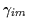).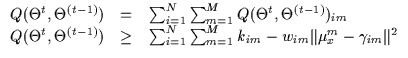(7.21)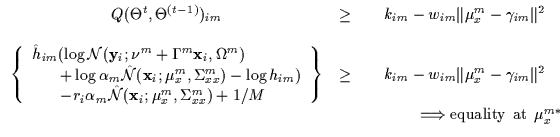(7.22)

Once again, the contact point for this parabola (i.e. the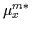) is the value for the previous model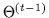or the mean of the gate before any iteration. Let us denote the previous parameters of the gate asand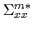. To facilitate the derivation, we can perform what is commonly called a whitening operation on the coordinate system for each gate. For each gate, we can consider that its previous parameters where zero-mean and identity covariance. This is done by applying an affine transformation to the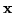-space (i.e. shifting the data points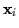appropriately). The data is translated by the mean and scaled by the inverse of the square root of the covariance matrix. In theory, nothing changes except that each gate observes affinely displaced data instead and it then seems to have a zero mean and identity covariance as its previous operating point. This whitening operation does not need to be done explicitly (i.e. numerically) but helps simplify the following derivation for the bound.

Thus, without loss of generality, we consider that each data point has been appropriately whitened for each gate. The result is that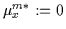and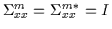. Using this assumption, we can compactly solve for the parameters of the bounding parabola (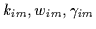). This is done by meeting the three requirements (contact, tangentiality and tightness). The results is shown in Equation 7.23.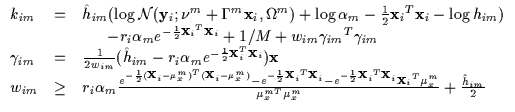(7.23)

As shown earlier, if a globally valid bound is desired, the value of wim must always be greater than the expression in Equation 7.23 no matter what the value of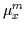. Thus we can find the minimum wim (i.e. wminim) for which this is true and form a parabolic bound for the Q function that is as 'tight' as possible. We shall now determine an efficient way to compute wminim without searching the high dimensional space ofeach iteration.

Note in the expression for wim above thatis used only in an inner product with itself (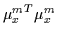) or in an inner product with the whitened data point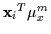. Thus, we can represent these two quantities regardless of the dimensionality of the vector space using at most two degrees of freedom: the magnitude ofand the cosine of the angle between the vectorsand. Let us now denote the new variables as follows: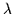is the magnitude of,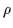is the magnitude ofand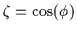is the cosine of the angle between the two vectors. We then compactly rewrite the bound as in Equation 7.24.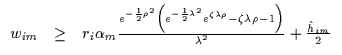(7.24)

The wim is now lower bounded by the right hand side which is a function of two free scalarsand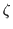. We wish to find the maximum of the lower bound at some value ofand. By taking derivatives of the right hand side with respect toand setting to 0, we find an extremum of the function at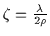. However, we also note thatand so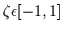. If we set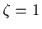we note that the this solution is always greater than the. So, the extremum we solved for was a minimum. Thus, the maximum occurs at the ends of the interval so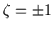. Therefore, we have the relationship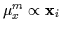. In other words, let us define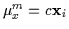. The expression for wim then simplifies into a function of 1 free variable (c) as in Equation 7.25.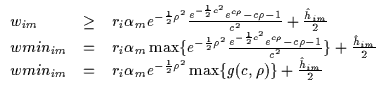(7.25)

For each value ofwe find the value of c which maximizes the value of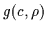. This is done offline (taking a few seconds) using Brent's method and the result is stored in a 1D lookup table (LUT). The values are shown in Figure 7.3(a) where we plot the max of the g function with respect to. Using this table, we can immediately compute the minimum wminim by looking up the corresponding value offor the current data point and subsequently compute the rest of the parabolic bound's parameters.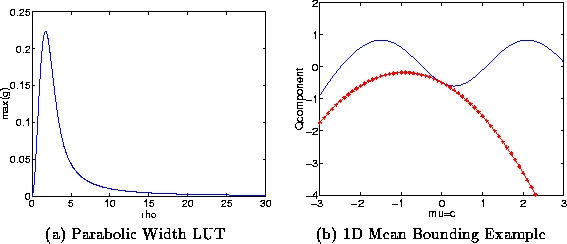An example of a parabolic bound is shown in Figure 7.3(b) under a sub-component of the Qfunction. This sub-component corresponds to a single data point as we vary one gate's uni-variate mean. Many such parabolic bounds are computed (one for each data point and gate combination) and are summed to bound the Q function in its entirety.

• Parabolic Piece-Wise Bound

For greater computational efficiency we compute the gradient of the Q on the mean to get an idea of the direction of steepest ascent. We can then know which general directionwill move towards for the next iteration. Due to whitening,starts off at the origin and moves from there. Thus, we know ifis moving close towardsor farther away from it. Equivalently, we know if c will be positive or negative. The above LUT (Figure 7.3(a)) returns the wminim which is valid for all possible choices of c. However, if we know the direction c is going to move along, we only need to consider half of the possibilities in using Brent's method to find the max of. Thus, we obtain two LUTs for c positive and for cnegative as shown in Figure 7.4(a). This typically gives us an even tighter bound since we only need to bound the Q function in regions of the landscape where the locus of the maximum could move to. Thus, we are effectively approximating the landscape with two parabolas, which are cut at c=0. One is a bound for the values at c<0 and one for c>0. Figure 7.4(b) depicts this piece wise parabolic bound on a sub-component of Q. The function being bounded is represented with a continuous line. One parabola is composed of 'x' symbols and is a valid bound to the right of zero. The other parabola is composed of 'o' symbols and is valid to the left of zero. The piece-wise bound is composed of these two parabolas spliced at the origin (their continuations are shown as dotted lines for visualization). Thus, if the gradient tells us that we will be moving in a direction of increasing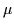, we should compute the parabola made of 'x' symbols. However, if we are moving to the left (because of the sum total influence of the other bounds and functions), we should use the parabola made of 'o' symbols which is a wider and tighter bound.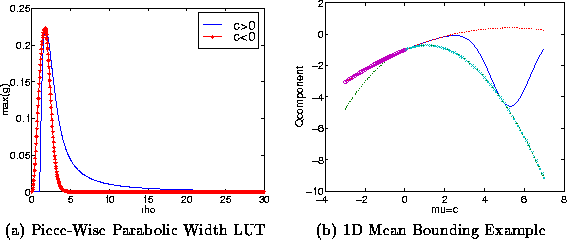To obtain a final answer for the update of the gate meanswe simply maximize the sum of parabolas which bounds the Q in its entirety. For M gates and N data points, we obtain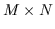parabolas each with its own (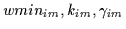). The update is in Equation 7.26.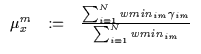(7.26)Next: Bounding Matrices: The Gate Up: CEM for Gaussian Mixture Previous: Bounding Scalars: The Gate
Tony Jebara
1999-09-15